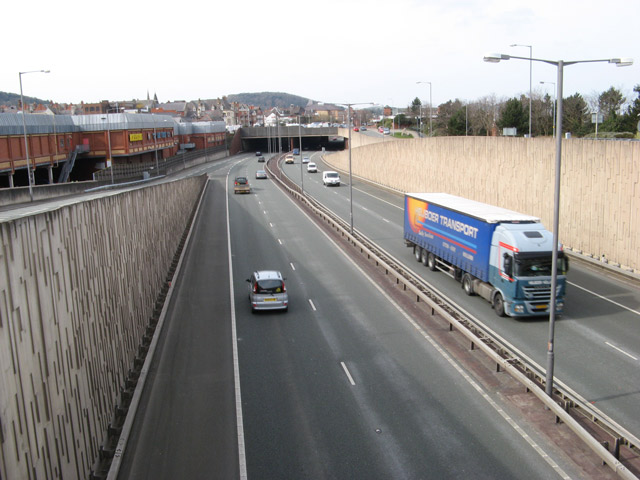# How Many Cars?(INPHO 2007)A car leaves point A for point B every ten minutes. The distance between A and B is 60 Km. The car travels at a speed of 60 km/hr.

What is the number of cars that a man driving from B to A will meet in route, if he starts from B simultaneously from one of the cars leaving A. The car from B also travels at 60 km/hr.

A question is a bit ambiguous and hence there can be two possible answers say (16,18). You have to figure out which are the two possible answers

HINT:-
1) Understand the question properly
2) Do it Graphically.

###### Image credit: Wikipedia Jonathan Wiklins
×

Problem Loading...

Note Loading...

Set Loading...